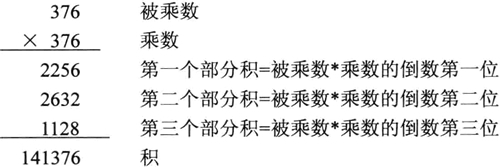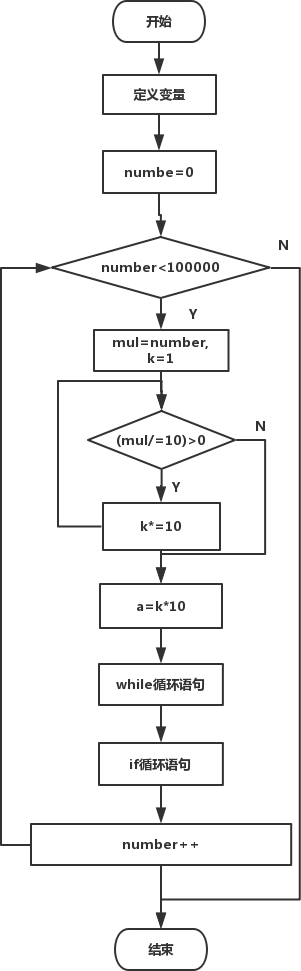## C语言求自守数（详解版）

• 内容
• 评论
• 相关

52 = 25    252 = 625    762 = 5776    93762 = 87909376

## 算法设计• 第一个部分积中：被乘数最后三位×乘数的倒数第一位。
• 第二个部分积中：被乘数最后二位×乘数的倒数第二位。
• 第三个部分积中：被乘数最后一位×乘数的倒数第三位。

#### 分离给定数中的最后几位

```int main ()
{
//...
while(k>0)
{
mul=( mul + ( number%(k*10) )*( number%b - nxuober%(b/10) ) )%a;
/* (部分积+截取被乘数的后N位*截取乘数的第M位），%a再截取部分积*/
k /= 10;  /*k为截取被乘数时的系数*/
b *= 10;
}
//...
return 0;
}``````#include<stdio.h>
int main()
{
long mul, number, k, a, b;
printf("It exists following automorphic nmbers small than 100000:\n");
for( number=0; number<100000; number++ )
{
for( mul=number, k=1; (mul/=10)>0; k*=10 );
/*由number的位数确定截取数字进行乘法时的系数k*/
a = k * 10;  /*a为截取部分积时的系数*/
mul = 0;  /*积的最后n位*/
b = 10;  /*b为截取乘数相应位时的系数*/
while(k>0)
{
mul=( mul + ( number%(k*10) )*( number%b - number%(b/10) ) )%a;
/*(部分积+截取被乘数的后N位*截取乘数的第M位)，%a再截取部分积*/
k /= 10;  /*k为截取被乘数时的系数*/
b *= 10;
}
if(number == mul)  /*判定若为自守数则输出*/
printf("%ld   ", number);
}
printf("\n");

return 0;
}```

It exists following automorphic nmbers small than 100000:
0   1   5   6   25   76   376   625   9376   90625

0条评论